Homework Help Question & Answers

11.3-10. Consider the following integer nonlinear programming problem Z = 18x1一斤+ 20x2 + 10x3, Maximize subject to 2x1 +4x2 + 3x3 S 11 and xi, x2, X3 are nonnegative integers Use dynamic progra...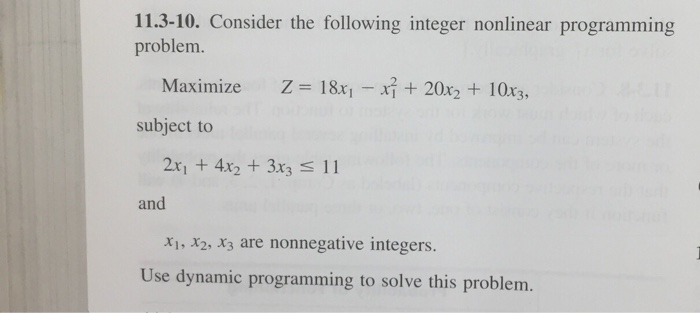11.3-10. Consider the following integer nonlinear programming problem Z = 18x1一斤+ 20x2 + 10x3, Maximize subject to 2x1 +4x2 + 3x3 S 11 and xi, x2, X3 are nonnegative integers Use dynamic programming to solve this problem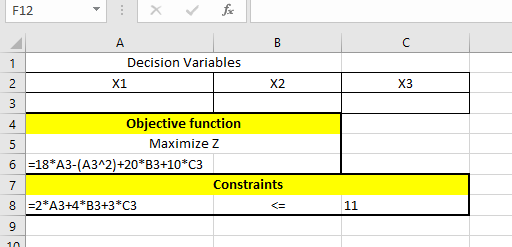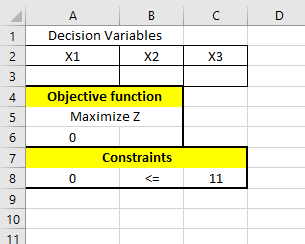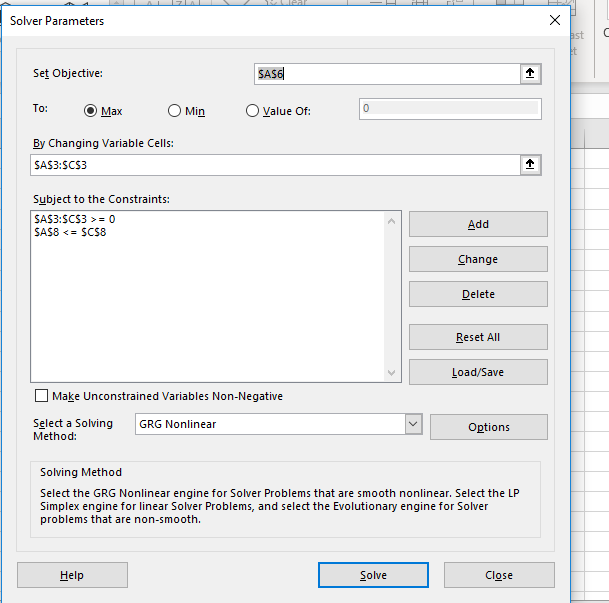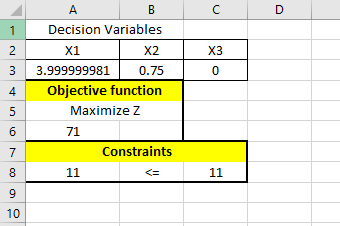Add Answer of: 11.3-10. Consider the following integer nonlinear programming problem Z = 18x1一斤+ 20x2 + 10x3, Maximize subject to 2x1 +4x2 + 3x3 S 11 and xi, x2, X3 are nonnegative integers Use dynamic progra...
More Homework Help Questions Additional questions in this topic.

• Problem 1 (20 pts) Consider the mathematical program max 3x1+x2 +3x3 s.t. 2x1 +x2 + x3 +x2 x1 + 2x2 + 3x3 +2xs 5 2x 2x2 +x3 +3x6-6 Xy X2, X3, X4, Xs, X620 Three feasible solutions ((a) through (c...

Need Online Homework Help?

Get FREE EXPERT Answers
WITHIN MINUTES
Related Questions